# RD Sharma Class 10 Ex 12.1 Solutions Chapter 12 Some Applications of Trigonometry

In this chapter, we provide RD Sharma Class 10 Ex 12.1 Solutions Chapter 12 Some Applications of Trigonometry for English medium students, Which will very helpful for every student in their exams. Students can download the latest RD Sharma Class 10 Ex 12.1 Solutions Chapter 12 Some Applications of Trigonometry pdf, Now you will get step by step solution to each question.

# Chapter 12: Some Applications of Trigonometry Exercise – 12.1

### Question: 1

A tower stands vertically on the ground. From a point on the ground, 20 m away from the foot of the tower, the angle of elevation of the top the tower is 60°. What is the height of the tower?

### Solution:

Given: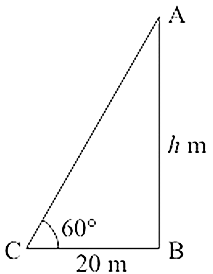Distance between point of observation and foot of tower = 20 m = BC

Angle of elevation of top of tower = 60° = θ

Height of tower H = AB

Now from fig ABC

ΔABC is a right angle triangle

### Question: 2

The angle of elevation of a ladder against a wall is 60° and the foot of the ladder is 9.5 m away from the wall. Find the length of the ladder.

### Solution:

Given:Distance between foot of the ladder and wall = 9.5 m

Angle of elevation θ = 60°

Length of the ladder = L = AC

Now figure forms a right angle triangle ABC

We know

∴ Length of the ladder (L) = 19 m

### Question: 3

A ladder is placed along a wall of a house such that its upper end is touching the top the wall. The foot of the ladder is 2 m away from the wall and the ladder is making an angle of 60° with the level of the ground. Determine the height of the wall.

### Solution: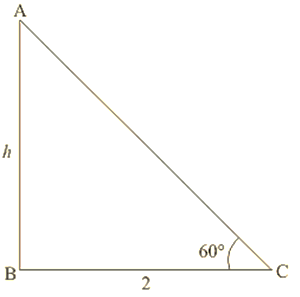Distance between foot of the ladder and wall = 2m = BC

Height of the wall H = AB

Now figure of ABC forms a right angle triangle

### Question: 4

An electric pole is 10 m high. A steel wire to top of the pole is affixed at a point on the ground to keep the pole up right. If the wire makes an angle of 45° with the horizontal through the foot of the pole, find the length of the wire.

### Solution:Height of the electric pole H = 10 m = AB

Angle made by steel wire with ground (horizontal) θ = 45°

Let length of wire = L = AC

If we represent above data in from of a figure then it forms a right angle triangle ABC.

Here

### Question: 5

A kite is flying at a height of 75 meters from the ground level, attached to a string inclined at 60° to the horizontal. Find the length of the string to the nearest meter.

### Solution:Given

Height of kite from ground = 75 m = AB

Inclination of string with ground θ = 60°

Length of string L = AC

If we represent the above data in form of figure as shown the it forms a right angle triangle ABC

Here,

### Question: 6

The length of a string between a kite and a point on a point on the ground is 90 meters. If the string makes an angle θ with the ground level such that tan θ = 15/8. How high is the kite? Assume that there is no slack in the string

### Solution: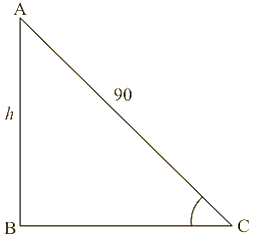Length of the string between point on ground and kite = 90 m

Angle made by string with ground is θ

And tan θ =15/8

θ = tan−1(frac158)

Height of the kite be ‘H’ m

If we represent the above data in figure as shown the it forms right angle triangle ABC

We have

In ΔABC, by Pythagoras theorem we have

H = 79.41

∴ Height of the kite from ground = 79.43 m

### Question: 7

A vertical tower stands on a horizontal place and is surmounted by a vertical flag staff. At a point on the plane 70 meters away from the tower, an observer notices that the angles of elevation of the meters away from the flag staff are respectively 60° and 45°. Find the height of the flag staff and that of the tower.

### Solution:

Given

Vertical tower is surmounted by flag staff.

Distance between tower and observer = 70 m = BC

Angle of elevation of top of tower α = 45°

Angle of elevation of top of flag staff β = 60°

Height of flag staff = h = AD

Height of tower = H = AB

If we represent the above data in the figure then it forms right angle triangles ΔABC and ΔBCD

When θ is angle in right angle triangle we know that

Now,

H = 70

∴ H =70

Again,

h = 70 (1.732-1)

h = 51.24 m

∴ h = 51.24 m

Height of tower = 80 m and height oh flag staff = 51.24 m

### Question: 8

A vertically straight tree, 15 m high; is broken by the wind in such a way that its top just touches the ground and makes an angle of 60° with the ground. At what height from the ground did the tree break?

### Solution: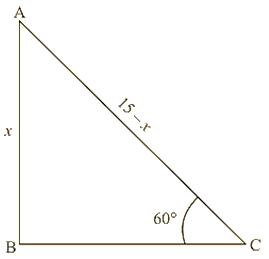Initial height of tree H = 15 m = AB

Let us assume that it is broken at point C.

Then given that angle made by broken part with the ground θ = 60°

Height from ground to broken points = h = BC

AB = AC + BC

H = AC + h

⟹ AC = (H – h) m

If we represent the above data in the figure as shown then it forms right angle triangle ABC from figure

Rationalizing denominator rationalizing factor of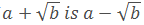∴ Height of broken points from ground### Question: 9

A vertical tower stands on a horizontal plane and is surmounted by a vertical flag staff of height 5 meters. At a point on the plane, the angles of elevation of the bottom and the top of the flag staff are respectively 30° and 60°. Find the height of the tower.

### Solution:

Height of the flag staff h = 5 m =AP

Angle of elevation of the top of flag staff = 60° = α

Angle of elevation of the bottom of the flagstaff = 60° = β

Let height of tower be ‘H’ m = AB

If we represent the above data in forms of figure then it forms a right angle triangle CBD in which ABC is included

In right angle triangle, if

Angle is θ then

Equating (1) and (2)

3H = H + 5

H = 2.5 m

Height of the tower = 2.5 m

### Question: 10

A person observed the angle of elevation of a tower as 30°. He walked 50 m towards the foot of the tower along level ground and found the angle of elevation of the top of the tower as 60°. Find the height of the tower.

### Solution:

Given

Angle of elevation of top of tour from first point of elevation (A) α = 30°

Let the walked 50 m from first point (A) to (B) then AB = 50 m

Angle of elevation from second point (B) β = 60°

Now let us represent the given data in form of figure

Then it forms ΔACDΔBCD in which ∠c = 90°

Let the height of the tower be ‘H’ m = CD

BC = x m.

If in a right angle triangle θ is the angle then

Equating (a) and (b)

### Question: 11

The shadow of a tower, when the angle of elevation of the sun is 45°, is found to be 10 m longer then when it was 60°. Find the height of the tower.

### Solution:

Let the length of the shadow of the tower when angle of elevation is 60° be x m = BC. Then according to problem

Length of the shadow with angle of elevation 45° is (10 + x)m = BD.

If we represent the above data in the form of figure then its forms a triangle ABD and triangle ABC where ∠B = 90°

Let height of the tower be ‘H’ m = AB

If in right angle triangle one of the angles is θ then

x + 10 = H

x = H – 10 … (b)

Substitute x = H – 10 in (a)

H = 23.66 m

### Question: 12

A parachute is descending vertically and makes angles of elevation of 45° and 60° at two observing points 100 m apart from each other on the left side of himself. Find the maximum height from which he falls and the distance of point where he falls on the ground from the just observation point.

### Solution:Let the parachute at highest point A. let C and D be points which are 100 m apart on ground where from then CD = 100 m

Angle of elevation from point C = 45° = α

Angle of elevation from point D = 60° = β

Let B be the point just vertically down the parachute

Let us draw figure according to above data then it forms the figure as shown in which ABC and ABD are two triangles

Maximum height of the parachute from the ground

AB = H m

Distance of point where parachute falls to just nearest observation point = x m

If in right angle triangle one of the included angles is θ then

From (a) and (b)

x = 136.6 m in (b)

H = √3 × 136.6 = 236.6m

Maximum height of the parachute from the ground

H = 236.6m

Distance between the two points where parachute falls on the ground and just the observation is x = 136.6 m

### Question: 13

On the same side of a tower, two objects are located. When observed from the top of the tower, their angles of depression are 45° and 60°. If the height of the tower is 150 m, find the distance between the objects.

### Solution:Height of the tower, H = AB = 150 m

Let A and B be two objects on the ground.

Angle of depression of object A'[∠A′ Ax] = β = 45° = ∠AA′B[Ax||A′B]

Angle of depression of object B'[∠xAB′] = α = 60° = ∠AB′B[Ax||A′B]

Let A’B’ = x;B’ B = y

If we represent the above data in form of a figure, Then it forms a right angle triangle with ∠B=90°

In any right angle triangle if one of the include angle is θ then

x + y = 150 … (b)

Substituting (a) in (b)

x = 63.4 m

Distance between object A’B’ = 63.4 m

### Question: 14

The angle of elevation of a tower from a point on the same level as the foot of the tower is 30°. On advancing 150 meters towards the foot of the tower, the angle of elevation of the tower becomes 60°. Show that the height of the tower is 129.9 m.

### Solution:

Angle of elevation of top tower from first point A, α = 30°

Let us advance through A to B by 150 m then AB = 150 m

Angle of elevation of top of tower from second point B, β = 60°

Let height of tower CD = H m

If we represent the above data in form of figure then it form a figure as shown with ∠D = 90°

In right angle triangle, one of included angle is θ

Substituting (b) in (a)

H = 129.9 m

∴ Height of the tower = 129.9 m

### Question: 15

The angle of elevation of the top of a tower from a point A on the ground is 30°. Moving a distance of 20 meters towards the foot of the tower to a point B the angle of elevation increases to 60°. Find the height of tower and the distance of the tower from the point A.

### Solution:

Angle of elevation of top of the tower from point A, α = 30°.

Angle of elevation of top of tower from point B, β = 60°.

Distance between A and B, AB = 20 m

Let height of tower CD = ‘h’ m

Distance between second point B from foot of the tower be ‘x’ m

If we represent the above data in form of figure then it form a figure as shown with ∠D = 90°

In right angle triangle, one of included angle is θ

Substituting (b) in (a)

x = 10 m

Height of the tower = 17.32 m

Distance of the tower from point A = (20 + 10) = 30 m

### Question: 16

From the top of a building 15 m high the angle of elevation of the top of tower is found to be 30°. From the bottom of the same building, the angle of elevation of the top of the tower is found to be 60°. Find the height of the tower and the distance between the building and the tower.

### Solution:

Let AB be the building and CD be the tower.

Height of the building is 15 m = h = AB

Angle of elevation of top of the tower from top of the building, α = 30°.

Angle of elevation of top of the tower from bottom of the building, β = 60°.

Distance between the tower and the building BD = x

Let height of the tower above building be ‘a’ m

If we represent the above data in form of figure then it form a figure as shown with ∠D = 90° also AX||BD, ∠AXC = 90°

Here ABDX is a rectangle

∴ BD = DX = ‘x’ m AB = XD = h = 15 m

In right triangle if one of the include angle is θ

Substituting (1) in (2)

x = 12.99 m

Height of the tower above ground = h + a

= 15 + 7.5

= 22.5 m

Distance between tower and building = 12.99 m

### Question: 17

On a horizontal plane there is a vertical tower with a flag pole on the top of the tower. At a point 9 m away from the foot of the tower the angle of elevation of the top and bottom of the flag pole are 60° and 30° respectively. Find the height of the tower and the flag pole mounted on it.

### Solution:Let AB be the tower and BC be the flagstaff on the tower

Distance of the point of observation from foot of the tower BD = 9 m

Angle of elevation of top of flagstaff, α = 60°

Angle of elevation of top of flagstaff, β = 30°

Let height of the tower = ‘x’ = AB

Height of the pole = ‘y’ = BC

If the above data is represented in form of figure a shown with ∠A = 90°

In right angle triangle, one of included angle is θ

x = 5.196 m … (b)

Substituting (b) in (a)

y = 9√3 – 3√3

y = 6√3

y = 10.392 m

∴ Height of the tower x = 5.196 m

Height of the tower pole = 10.392 m

### Question: 18

A tree breaks due to storm and the broken part bends so that the top of the tree touches the ground making an angle of 30° with the ground. The distance between the foot of the tree to the point where the top touches the ground is 8 m. Find the height of the tree.

### Solution: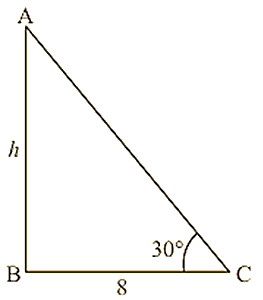Let the initial height be AB

Let us assumed that the tree is broken at point C

Angle made by the broken part CB’ with ground is 30° = θ

Distance between foot of tree to point where it touches the ground B’A = 8 m

Height of the tree = h = AC + CB’ = AC + CB

The above information is represent in the form of figure as shown

### Question: 19

From a point P on the ground the angle of elevation of a 10 m tall building is 30°. A flag is hoisted at the top of the building and the angle of elevation of the flag staff from P is 45°. Find the length of the flag staff and the distance of the building from the point P.

### Solution:

Let AB be the tower and BD be the flag staff

Angle of elevation of top of the building from P α = 30°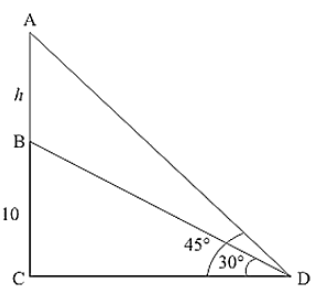AB = height of the tower = 10 m

Angle of elevation of top of flag staff from P β = 45°

Let height of the flagstaff BD = ‘a’ m

The above information is represented in the form of figure as shown

with ∠A = 90°

In a right angle triangle if one of included angle is θ

10 + a = AP

a = 17.32 -10 m

a = 7.32 m

Height of the flag staff ‘a’ = 7.32 m

Distance between P and foot of the tower = 17.32 m

### Question: 20

A 1.6 m tall boy stands at a distance of 3.2 m from a lamp post and casts a shadow of 4.8 m on the ground. Find the height of the lamp post by using

(i) trigonometric ratio

(ii) properties of similar triangles

### Solution:Let AC be the lamp post of height ‘h’

We assume that ED = 1.6 m, BE = 4.8 m and EC = 3.2

We have to find the height of the lamp post

Now we have to find the height of the lamp post using similar triangles

Since triangle BDE and triangle ABC are similar

Again we have to find the height of lamp post using trigonometric ratio

tan θ = 1.6/4.8

tan θ = 13

Again In ΔABC

Hence the height of the lamp post is h = 8/3 m

### Question: 21

From a point on the ground the angle of elevation of the bottom and top of a transmission lower fixed at the top of 20 m high building are 45° and 60° respectively. Find the height of the transmission tower.

### Solution:Given

Height of building = 20 m = AB

Let height of tower above building = ‘h’ = BC

Height of tower + building = (h + 20) m [from ground] = CA

Angle of elevation of bottom of tour, α = 45°

Angle of elevation of top of tour, β = 60°

Let distance between tower and observation point = ‘x’m

The above data is represented in the form of figure as shown is

If one of the include angle in right angle triangle is θ

x = 20 m

h = 20(1.73 − 1) m

h = 20 x .732

h = 14.64 m

### Question: 22

The angle of depression of the top and bottom of 8 m tall building from the top of a multistoried building are 30° and 45°. Find the height of the multistoried building and the distance between the two buildings.

### Solution:Let height of multistoried building ‘h’ m = AB

Height of the tall building = 8 m = CD

Angle of depression of top of the tall building, α = 30°

Angle of depression of bottom of the tall building, β = 30°

Distance between the two buildings = ‘x’ m = BD

Let AO = s

AB = AO + BO but BO = CD           [∴ AOCD is rectangle]

AB = (s + 8) m

The above information is represented in the form of figure as shown

If in right angle triangle one of the included angle is θ

In ΔAOC

In ΔABD

s + 8 = x … (2)

Substituting (a) in (2)

s + 8 = s√3

s(√3 – 1) = 8

### Question: 23

A statue 1.6 m tall stands on the top of a pedestal. From a point on the ground, angle of elevation of the top of the statue is 60° and from the same point the angle of elevation of the top of the pedestal is 45°. Find the height of the pedestal.

### Solution:

Let the height of the pedestal be ‘h’ m

Height of the statue = 1.6 m

Angle of elevation of the top of the statue α = 30°

Angle of elevation of the top of the pedestal β = 30°

The above data is represented in the form of figure as shown.

If in right angle triangle one of the included angle is θ

DC = ‘h’ m …. (a)

From (a) and (b)

### Question: 24

A T.V. tower stands vertically on a bank of a river of a river. From a point on the other bank directly opposite the tower, the angle of elevation of the top of the tower is 60°. From a point 20 m away this point on the same bank, the angle of elevation of the top of the tower is 30°. Find the height of the tower and the width of the river.

### Solution:Let AB be the T.V tower of height ‘h’ m on the bank of river and ‘D’ be the point on the opposite side of the river. An angle of elevation at the top of the tower is 30°

Let AB = h and BC = x

Here we have to find height and width of the river.

The above data is represented in the form of figure as shown.

In ΔACB

Again in ΔDBA,

x = 10

Hence the height of the tower is 10√3 m and width if the river is 10 m.

### Question: 25

From the top of a 7 m high building, the angle of elevation of the top of a cable is 60° and the angel of depression of its foot is 45°. Determine the height of the tower.

### Solution:

Given

Height of the building = 7 m = AB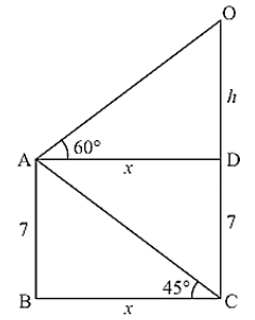Height of the cable tower = ‘H’ m = CD

Angle of elevation of the top of the building α = 60°

Angle of elevation of the bottom of the building β = 45°

The above data is represented in form of figure as shown

Let CX = ‘x’ m

CD = DX + XC = 7 m + ‘x’ m

= x + 7m

### Question: 26

As observed from the top of a 75 m tall lighthouse, the angles of depression of two ships are 30° and 45°. If one ship is exactly behind the other on the same side of the lighthouse, find the distance between the two ships.

### Solution:

Given;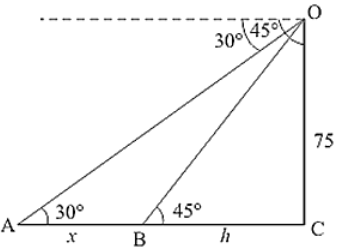Height of the lighthouse = 75m = ‘h’ m = AB

Angle of depression of ship 1, α = 30°

Angle of depression of bottom of the tall building, β = 45°

The above data is represented in form of figure as shown

Let distance between ships be ‘x’ m = CD

If in right angle triangle one of the included angle is θ

BC = 75 … (2)

Substituting (2) in (1)

∴ distance between ships = ‘x’ m

### Question: 27

The angle of elevation of the top of the building from the foot of the tower is 30° and the angle of the top of the tower from the foot of the building is 60°. If the tower is 50 m high, find the height of the building.

### Solution:

Angle of elevation of top of the building from foot of tower = 30° = αAngle of elevation of top of the building from foot of tower = 60° = β

Height of the tower = 50 m = AB

Height of building = ‘h’ m = CD

The above data is represented in the form of figure as shown

In right triangle if one of the included angle is θ

h = 50/3

∴  height of the building = 503/m

### Question: 28

From a point on a bridge across a river the angle of depression of the banks on opposite side of the river are 30° and 45° respectively. If the bridge is at the height of 30 m from the banks, find the width of the river.

### Solution:

Height of the bridge = 30 m [AB]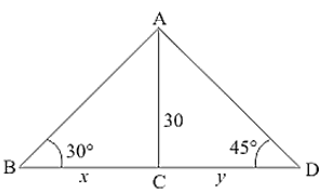Angle of depression of bank 1 i.e, α = 30° B1

Angle of depression of bank 2 i.e, β = 30° B2

Given banks are on opposite sides

Distance between banks B1B2 = BB1 + BB2

The above data is represented in the form of figure as shown

In right angle triangle, if one of the included angle is θ

B2B = 30 m

B1B2 = BB1 + BB2

Distance between banks

### Question: 29

A man sitting at a height of 20 m on a tall tree on a small island in the middle of a river observes two poles directly opposite to each other on the two banks of the river and in line with foot of tree. If the angles of depression of the feet of the poles from a point at which the man is sitting on the tree on either side of the river are 60° and 30° respectively. Find the width of the river.

### Solution:

Height of the tree AB = 20 mAngle of depression of the pole 1 feet α = 60° B1

Angle of depression of the pole 2 feet β = 30° B1

B1C1 be one pole and B2C2 be the other pole

Given poles are on opposite sides.

Width of the river = B1B2

= B1B + BB2

The above data is represented in the form of figure as shown

In right angle triangle, if one of the included angle is θ

B1B2 = B1B + BB2

Width of the river = 80/√3 m

### Question: 30

A vertical tower stands on a horizontal plane and is surmounted by a flag staff of height 7m. From a point on the plane, the angle of elevation of the bottom of flag staff is 30° and that of the top of the flag staff is 45°. Find the height of the tower.

### Solution:

Given

Height of the flag staff = 7 m = BC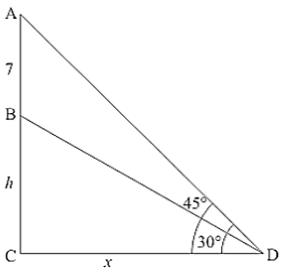Let height of the tower = ‘h’ m = AB

Angle of elevation of top of the bottom of the flagstaff α = 30°

Angle of elevation of top of the top of the flagstaff β = 45°

Points of desecration be ‘p’

The above data is represented in the form of figure as shown

In right angle triangle, if one of the included angle is θ

From (1) and (2)

Height of the tower = 3.5(√3 + 1) m.

### Question: 31

The length of the shadow of a tower standing on level plane is found to be 2x meters longer when the sun’s attitude is 30° than when it was 30°. Prove that the height of tower is x(√3 + 1)meters.

### Solution:

Let

Length of shadow be ‘a’ m [BC] when sun altitude be α = 45°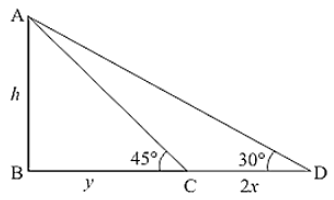Length of shadow be (2x + a) m [BD] when sun altitude be β = 30°

Let height of tower be ‘h’ m = AB

The above data is represented in the form of figure as shown

In right angle triangle one of the included angle is θ

h = a … (1)

Substituting (1) in (2)

Height of the tower = x√3 + 1) m

### Question: 32

A tree breaks due to storm and the broken part bends so that the top of the tree touches the ground making an angle of 30° with the ground. The distance from the foot of the tree to the point where the top touches the ground is 10 meters. Find the height of the tree.

### Solution:

Let AB be the height of the tree and it is broken at point C and top touches ground at B’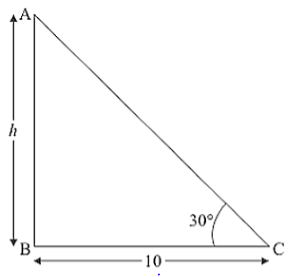Angle made by the top α = 30°

Distance from foot of tree from point where it touches ground = 10 m

The above data is represented in form of figure as shown

Height of the tree = AB = AC + CB

= AC + CB’

In right angle triangle one of the included angle is θ

AB = CA + CB’

Height of tree = 10/√3 m

### Question: 33

A balloon is connected to a meteorological ground station by a cable of length 215 m inclined at 60° to the horizontal. Determine the height of the balloon from the ground. Assume that there is no slack in the cable.

### Solution:

Length of the cable connected to balloon = 215 m [CB]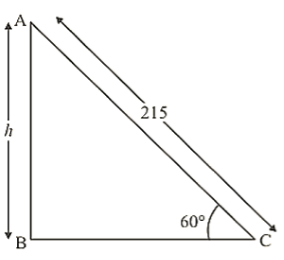Angle of inclination of cable with ground α = 60°

Height of the balloon from ground = ‘h’ m = AB

The above data is represented in from of figure as shown

In right angle triangle one of the included angle is θ

Height of the balloon from ground = 107.5√3 m

### Question: 34

Two men on either side of the cliff 80 m high observe the angles of elevation of the top of the cliff to be 30° and 60° respectively. Find the distance between the two men.

### Solution:

Height of cliff = 80 m = AB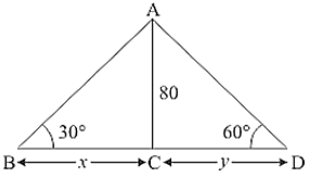Angle of elevation from Man 1, α = 30° [M1]

Angle of elevation from Man 2, β = 60° [M2]

Distance between two men = M1M2 = BM1 + BM2

The above information is represented in form of figure as shown

In right angle triangle one of the included angle is θ

M1M2 = M1B + BM2

Distance between men = 320√3 meters

### Question: 35

Find the angle of elevation of the sun (sun’s altitude) when the length of the shadow of a vertical pole is equal to its height.

### Solution:

Let

Height of the pole = ‘h’ m = sun’s altitude from ground length of shadow be ‘L’

Given that L = h.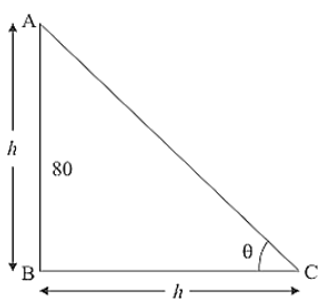Angle of elevation of sun’s altitude be θ

The above data is represented in from of figure as shown

In right angle triangle one of the included angle is θ

θ = tan−1(1) = 45°

Angle of sun’s altitude is 45°

### Question: 36

A man standing is on the deck of a ship, which is 8 m above water level. He observes the angle of elevation of the top of a hill as 60° and the angle of depression of the base of the hill as 30°. Calculate the distance of the hill from the ship and the height of the hill.

### Solution:

Height of the ship above water level = 8 m = AB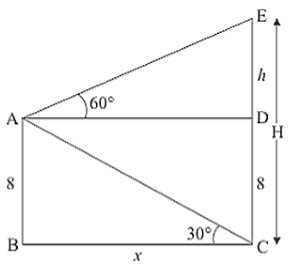Angle of elevation of top of cliff (hill) α = 60°

Angle of depression of the bottom of hill β = 30°

Height of the hill = CD

Distance between ship and hill = AX

Height of the hill above ship = CX = ‘a’ m

Height of hill = (a + 8) m

The above data is represented in form of figure as shown

In right angle triangle if one of the included angle is θ

a = 24 m

AX = 8√3 m

∴ Height of the cliff hill = (24 + 8) m = 32 m

Distance between hill and ship 8√3 m

### Question: 37

The angles of depression of two ships from the top of a light house and on the same side of it are found to be 45° and 30° respectively. If the ships are 200 m apart, find the height of the light house.

### Solution:

Height of the light house AB = ‘h’ metersLet S1 and S2 be ships

Distance between ships S1S2 = 200 m

Angle of depression of S1(α = 30°)

Angle of depression of S2(β = 45°)

The above data is represented in form of form of figure as shown

In ΔABS2

BS2 = h … (1)

In ΔABS1

Subtracting (1) from (2)

Height of the light house = 273.2 meters

### Question: 38

The angles of elevation from the top of a tower from two points at distance of 4 m and 9 m from the base of the tower and in the same straight line with it are complementary. Prove that the height of the tower is 6 m.

### Solution:

Height of the tower AB = ‘h’ metersLet point C be 4 meters from B.

Angle of elevation is α given point D is 9 meters from B, Angle of elevation is β

Given α, β are complementary, α + β = 90°

⟹ β = 90° – α

Required to prove that h = 6 meters

The above data is represented in the form of figure as shown

In ΔABC

In ΔABD

h = 9 tanα … (2)

Multiply (1) and (2)

h × h = 4 tan α × 9 cot α

h2 = 36 (tan α cot α)

h = √36 = 6 m

Height of the tower = 6 meters

### Question: 39

From the top of a 50 m high tower, the angles of depression of the top and bottom of a pole are observed to be 45° and 60° respectively. Find the height of the pole.

### Solution:

AB = height of the tower = 50 m.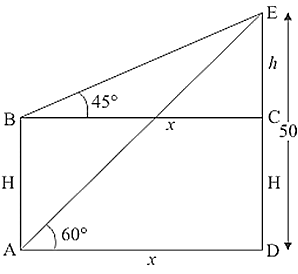CD = height of the pole

Angle of depression of top of building α = 45°

Angle of depression of bottom of building β = 60°

The above data is represented in the form of figure as shown

In right angle triangle if one of the included angle is θ

Distance between the pole and tower = 50/√3 m

### Question: 40

The horizontal distance between two trees of different heights is 60 m. the angles of depression of the top of the first tree when seen from the top of the second tree is 45°. If the height of the second tree is 80 m, find the height of the first tree.

### Solution:

Distance between the trees = 60 m [BD]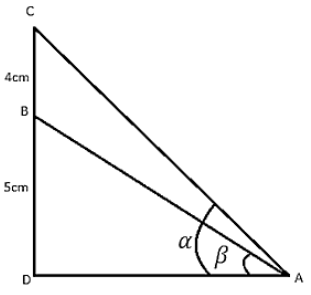Height of second tree = 80 m [CD]

Let height of the first tree = ‘h’ m [AB]

Angle of depression from second tree top to first tree top α = 45°

The above data is represented in form of figure as shown

In right angle triangle if one of the included angle is θ

Draw CX ⊥ AB

CX = BD = 60 m

XB = CD = AB – AX

tan α = AX/CX

tan 45° = AX/60

AX = 60 m

XB = CD = AX – AX

= 80 – 60

= 20 m

Height of the second tree = 80 m

Height of the first tree = 20 m

### Question: 41

A flag staff stands on the top of a 5 m high tower. From a point on the ground, angle of elevation of the top of the flag staff is 60° and from the same point, the angle of elevation of the top of the tower is 45°. Find the height of the flag staff.

### Solution:

Height of tower = AB = 5Height of flag staff BC = ‘h’ m

Angle of elevation of top of flagstaff α = 60°

Angle of elevation of bottom of flagstaff β = 45°

The above data is represented in form of figure as shown

In ΔABC

tan β = AB/DA

tan 45° = 5/DA

DA = 5 cm

tan α = AC/DA

height of the flagstaff = 3.65 mts

### Question: 42

As observed from the top of a 150 m tall light house, the angles of depression of two ships approaching it are 30° and 45°. If one ship is directly behind the other, find the distance between the two ships.

### Solution:

Height of the light house AB = 150 meters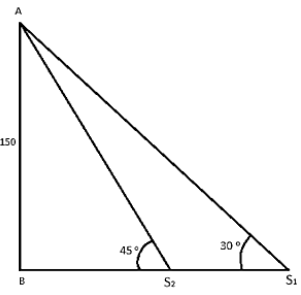Let S1 and S2 be two ships approaching each other

Angle of depression of S1, α = 30°

Angle of depression of S2, β = 45°

Distance between ships = S1S2

The above data is represented in the form of figure as shown

In ΔABS2

tan β = AB/BS2

tan45° = 150/BS2

BS2 = 150 m

In ΔABS1

tan α = ∆ABS1

tan 30° = 150/BS1

### Question: 43

The angle of elevation of the top of a rock form the top and foot of a 100 m high tower are respectively 30° and 45°. Find the height of the rock

### Solution:

Height of the tower AB = 100 mHeight of rock CD = ‘h’ m

Angle of elevation of the top of rock from top of the tower α = 30circ

Angle of elevation of the top of rock from bottom of tower β = 60°

The above data is represented in the form of figure as shown

Draw AX ⊥ CD

XD = AB = 100 m

XA = DB

In ΔCXA

In ΔCBD

DB = 100 + CX … (2)

From (1) and (2)

### Question: 44

A straight highway leads to the foot of a tower of height 50 m. From the top of the tower, the angles of depression of two cars standing on the highway are 30° and 60° respectively. What is the distance between the two cars and how far is each car from the tower?

### Solution:

Height of the tower AB = 50 m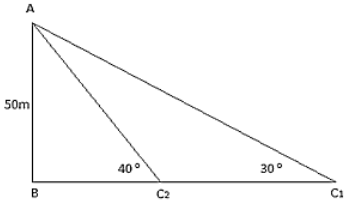C1 and C2 be two cars

Angles of depression of C1 from top of the tower α = 30°

Angles of depression of C2 from top of the tower β = 60°

Distance between cars C1 and C2

The above data is represented in form of figure as shown

In ΔABC2

In ΔABC1

C1 and C2 = BC1 − BC2

### Question: 45

From the top of a building AB = 60 m, the angles of depression of the top and bottom of a vertical lamp post CD are observed to be 30° and 45° respectively. Find

(i) The horizontal distance between AB and CD

(ii) The height of the lamp post

(iii) The difference between the heights of the building and the lamp post.

### Solution:

Height of building AB = 60 m

Height of lamp post CD = ‘h’ m

Angle of depression of top of lamp post from top of building α = 30°

Angle of depression of bottom of lamp post from top of building β = 60°

The above data is represented in form of figure

Draw DX⊥AB, CX = AC, CD = AX

In ΔBDX

In ΔBCA

From (1) and (2)

60 – h = 20

h = 40m

Height of the lamp post = 40m

Distance between lamp post buildings AC = 20√3 m

Difference between heights of building and lamp post BX = 60 – h ⟹ 60 – 40 = 20 m.

### Question: 46

Two boats approach a light house in mid sea from opposite directions. The angles of elevation of the top of the light house from two boats are 30° and 45° respectively. If the distance between the two boats is 100m, find the height of the light house.

### Solution:

Let B1 be boat 1 and B2 be boat 2Height of light house = ‘h’ m = AB

Distance between B1B2 = 100 m

Angle of elevation of A from B1α = 30°

Angle of elevation of B from B2β = 45°

The above data is represented in the form of figure as shown

Here In ΔABB1

In ΔABB2

h = BB2 … (2)

### Question: 47

The angle of elevation of the top of a hill at the foot of a tower is 60° and the angle of elevation of the top of the tower from the foot of the hill is 30°. If tower is 50 m high, what is the height of the hill?

### Solution:

Height of tower AB = 50 m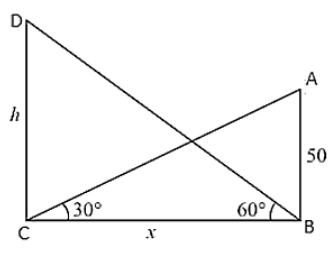Height of hill CD = ‘h’ m.

Angle of elevation of top of the hill from foot of the tower = α = 60°

Angle of elevation of top of the tower from foot of the hill = β = 30°

The above data is represented in the form of figure as shown

From figure

In ΔABC

tan 30° = AB/BC

In ΔBCD

CD = 150 m

Height of hill = 150 m.

### Question: 48

A fire in a building B is reported on telephone to two fire station P and Q 20 km apart from each other on a straight road. P observes that the fire is at an angle of 60° to the road and Q observes that it is at an angle of 45° to the road. Which station sends its team and how much will this team have to travel?

### Solution:

Let AB be the buildingAngle of elevation from point P [fire station 1] α = 60°

Angle of elevation from point Q [fire station 1] β = 45°

Distance between fire station PQ = 20 km

The above data is represented in form of figure as shown

In right angle triangle if one of the included angle is θ

AQ = AB … (2)

Station 1 should send its team and they have to travel 7.32 km

### Question: 49

A man on the deck of a ship is 10 m above the water level. He observes that the angle of elevation of the top of a cliff is 45° and the angle of depression of the base is 30°. Calculate the distance of the cliff from the ship and the height of the cliff.

### Solution:

Height of the ship from water level = 10 m = AB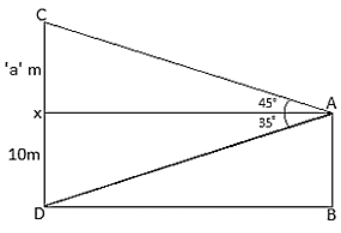Angle of elevation of top of the cliff α = 45°

Angle of elevation of bottom of the cliff β = 30°

Height of the cliff CD = ‘h’ m

Distance of the ship from foot of the tower cliff.

Height of cliff above ship be ‘a’ m

Then height of cliff = DX + XC = (10 + a) m

The above data is represented in form of figure as shown

In right angle triangle, if one of the included angle is θ

AX = ‘a’ cm

### Question: 50

There are two temples one on each bank of a river opposite to one other. One temple is 50 m high. Form the top of this temple, the angles of depression of the top and foot of the other temple are 30° and 60° respectively. Find the width of the river and the height of the other temple.

### Solution:

Height of the temple 1 (AB) = 50 mAngle of depression of top of temple 2, α = 30°

Angle of depression of bottom of temple 2, β = 60°

Height of the temple 2 (CD) = ‘h’ m

Width of the river = BD = ‘x’ m

The above data is represented in from of figure as shown

In right angle triangle if one of the included angle is θ

Here BD = CX, CD = BX

### Question: 51

The angle of elevation of an airplane from a point on the ground is 45°. After a flight of 15 seconds, the elevation changes to 30°. If the airplane is flying at a height of 3000 meters, find the speed of the airplane.

### Solution:

Let the airplane travelled from A to B in 15 sec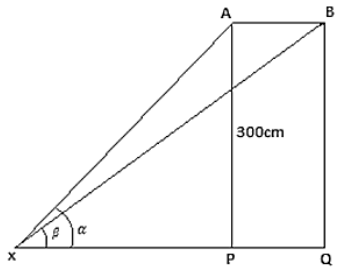Angle of elevation of point A α = 45°

Angle of elevation of point B β = 30°

Height of the airplane from ground = 3000 meters

= AP = BQ

Distance travelled in 15 seconds = AB = PQ

Velocity (or) speed = distance travelled time

If the above data is represented is form of figure as shown

In right angle triangle if one of the included angle is θ

XP = 3000 m

2000 × 0.732

146.4 m/sec

Speed of the airplane = 146.4 m/sec

### Question: 52

An airplane flying horizontally 1 km above the ground is observed at an elevation of 60°. After 10 seconds, its elevation is observed to be 30°. Find the speed of the airplane in km/hr.

### Solution:

Let airplane travelled from A to B in 10 seconds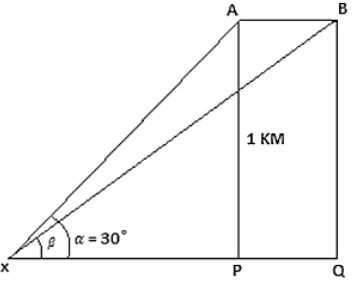Angle of elevation of point A α = 60°

Angle of elevation of point B β = 30°

Height of the airplane from ground = 1 km = AP = BQ

Distance travelled in 10 seconds = AB = PQ

The above data is represented in form of figure as shown

In right angle triangle if one of the included angle is θ

### Question: 53

A tree standing on a horizontal plane is leaning towards east. At two points situated at distance a and b exactly due west on it, the angles of elevation of the top are respectively α and β. Prove that the height of the top from the ground is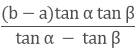### Solution:

AB be the tree leaning eastFrom distance ‘a’ m from tree, angle of elevation be α at point P.

From distance ‘b’ m from tree, angle of elevation be β at point Q.

The above data is represented in the form of figure as shown

In right angle triangle if one of the included angle is θ

Draw AX⊥QB let BX = ‘a’ m

x + a = AX cot α … (1)

x + b = AX cot β … (2)

Subtracting (1) from (2)

(x + b) – (x + a) = AX cot − AX cot α

### Question: 54

The angle of elevation of a stationary cloud from a point 2500 m above a lake is 15° and the angle of depression of its reflection in the lake is 45°. What is the height of the cloud above the lake level?

### Solution:

Let the cloud be at height PQ as represented from lake level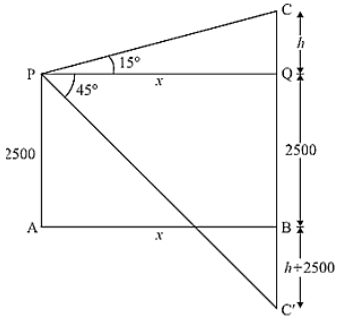From point x, 2500 meters above the lake angle of elevation of top of cloud α = 15°

Angle of depression of shadow reflection in water β = 45°

Here above data is represented in form of figure as shown

In right angle triangle if one of the included angle is θ

tan15° = AQ/AY

0.268 = h/AY

AY = h/0.268 … (1)

tan 45° = AB’/AY

AY = x + (h + x)

AY = h + 2x … (2)

From (1) and (2)

h/0.268 = h + 2x

3.131h – h = 2 × 2500

h = 1830.8312

Height of the cloud above lake = h + x

= 1830.8312 + 2500

= 4330.8312 m

### Question: 55

If the angle of elevation of a cloud from a point h meters above a lake is α and the angle of depression of its reflection in the lake be β, prove that the distance of the cloud from the point of observation is

### Solution:

Let x be point ‘h’ meters above lake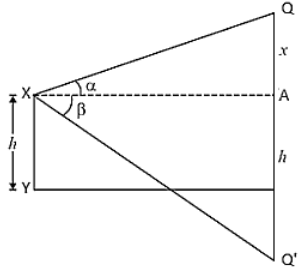Angle of elevation of cloud from x = α

Angle of depression of cloud reflection in lake = β

Height of the cloud from lake = PQ

PQ’ be the reflection then PQ’ = PQ

Draw XA + PQ, AQ = ‘x’ m, AP = ‘h’ m

Distance of cloud from point of observation is XQ.

The above data is represented in form of figure as shown

In ΔAQX

tanα = AQ/AX

tanα = x/AX … (a)

In ΔAXQ

Tan β = AQ’/AX

Subtracting (1) from (2)

tan β – tan α = 2h/AX

In ΔAQX

cos α = AX/XQ

QX = AX secα

### Question: 56

From an airplane vertically above a straight horizontal road, the angle of depression of two consecutive mile stones on opposite side of the airplane are observed to be α and β. Show that the height in miles of airplane above the road is given by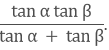### Solution:

Let PQ be the height of airplane from ground x and y be two mile stones on opposite side of the airplane xy = 1 mile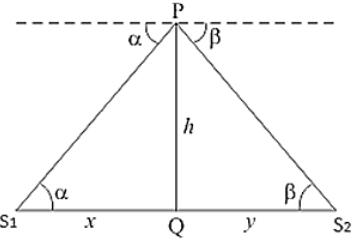Angle of depression of x from p = α

Angle of depression of y from p = β

The above data is represented in the form of figure as shown

In right angle triangle if one of the included angle is θ

In ΔPXQ

tan α = PQ/XQ

XQ = PQ/tanα … (1)

In ΔXQY

tan β = PQ/QY

QY = PQ/tanβ … (2)

XQ + QY = PQ/tanβ + PQ/tanα

XY = PQ(1/tanβ + 1/tanα)

### Question: 57

PQ is a post given height ‘a’ m and AB is a tower at same distance. If α and β are the angles of elevation of B, the top of the tower, at P and Q respectively. Find the height of the tower and its distance from the post.

### Solution:

PQ is part height = ‘a’ m AB is tower height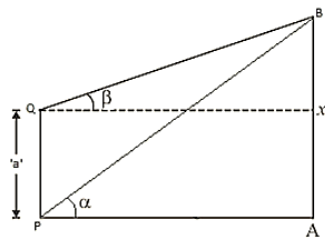Angle of elevation of B from P = α

Angle of elevation of B from Q = β

The above data is represented in gorm of figure as shown

In right angle triangle if one of the included angle is θ

QX⊥AB, PQ = AK

In ΔABQ

tan β = BX/QX

In ΔBPA

tanα = AB/AP

tanα = AB/QX … (2)

Dividing (1) by (2)

### Question: 58

A ladder rest against a wall at an angle α to the horizontal. Its foot is pulled away from the wall through a distance a, so that it slides a distance b down the wall making an angle β with the horizontal. Show that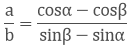### Solution:

Let AB be the ladder initially at an inclination α to groundWhen its foot is pulled through distance ‘a’ let BB’ = ‘a’ m and AA’ = ‘b’ m

New angle of elevation from B’ = B the above data is represented in form of figure as shown

Let AP⊥ ground B’P, AB = A’B’

A’P = x        BP = y

In ΔABP

sin α = AP/AB

cos α = BP/AB

cos α = y/AB …. (2)

In ΔA’B’P

sin β = A’P/A’B’

sin β = x/AB … (3)

cos β = B’P/A’B’

Subtracting (3) from (1)

sin α − sin β = b/AB

Subtracting (4) from (2)

cos β − cos α = a/AB

### Question: 59

A tower subtends an angle α at a point A in the plane of its base and the angle if depression of the foot of the tower at a point b meters just above A is β. Prove that the height of the tower is b tan α cot β.

### Solution:

Let height of the tower be ‘h’ m = PQ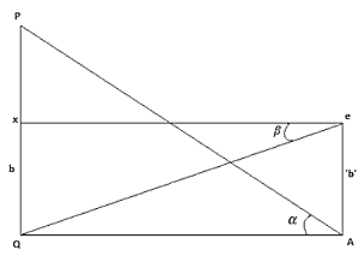Angle of elevation at point A on ground = α

Let B be the point ‘b’ m above the A

Angle of depression of foot of the tower from B = β

The above data is represented in form of figure as shown

Let BX⊥P, AB = A’B’

In ΔPBX

tanα = PQ/BX … (1)

In ΔQBX

tanβ = QX/BX … (2)

Dividing (1) by (2)

### Question: 60

An observer, 1.5 m tall, is 28.5 m away from a tower 30 m high. Determine the angle of elevation of the top of the tower from his eye.

### Solution:

Height of observer = AB = 1.5 m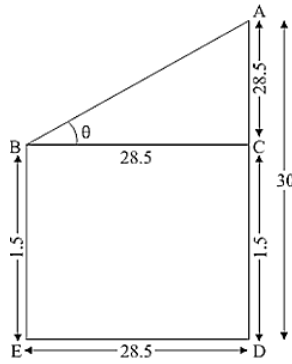Height of tower = PQ = 30 m

Height of tower above the observer eye = 30 – 1.5

QX = 28.5 m

Distance between tower and observer XB = 28.5 m

θ be angle of elevation of tower top from eye

The above data is represented in the form of figure

θ = tan−1(1) = 45°

Angle of elevation = 45°

### Question: 61

A carpenter makes stools for electricians with a square top of side 0.5 m and at a height of 1.5 m above the ground. Also each leg is inclined at an angle of 60° to the ground. Find the length of each leg and also the lengths of two steps to be put at equal distance.

### Solution:

Let AB be the height of stool = 1.5 m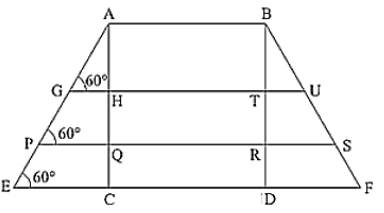Let P and Q be equal distance then AP = 0.5. AQ = 1 m

The above data is represented in form of figure as shown

BC = length of leg

sin 60° = AB/BC

Draw PX⊥AB, QZ⊥AB, XY⊥CA, ZW⊥CA

sin 60° = XY/XC

XC = 1.1077 m

sin 60° = ZW/CZ

CZ = 1.654 m

### Question: 62

A boy is standing on the ground and flying a kite with 100 m of string at an elevation of 30°. Another boy is standing on the roof of a 10 m high building and is flying his kite at an elevation of 45°. Both the boys are on opposite sides of both the kites. Find the length of the string that the second boy must have so that the kites meet.

### Solution:

For boy 1Length of the string AB = 100 m

Angle made by string with ground = α = 30°

For boy 2

Height of the building CD = 10 m

Angle made by string with building top = β = 45°

Length of the kite thread of boy 2 if both the kites meet must be ‘DB’

The above data is represented in form of figure as shown

Draw BX⊥AC, YD⊥BC

In ΔABX

tan 30° = BX/AX

sin 30° = BX/AB

1/2 = BX/100

BX = 50 m

BY = BX – XY = 50 – 10 = 40 m

In ΔBYD

sin 45° = BY/BD

Length of thread or string of boy 2 = 40√2

### Question: 63

From the top of a light house, the angles of depression of two ships on the opposite sides of it are observed to be α and β. If the height of the light house be h meters and the line joining the ships passes through the foot of the light house, show that the distance### Solution:

Height of light house = ‘h’ meters = AB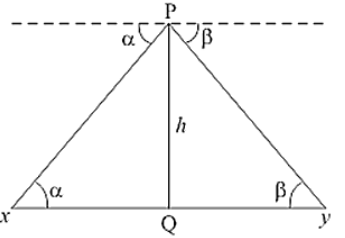S1 and S2 be two ships on opposite sides of the light house

Angle of depression of S1 from top of light house = α

Angle of depression of S2 from top of light house = β

Required to prove that

The above data is represented in the form of figure as shown

In ΔABS1

In ΔABS2

tan β =AB/S2B

S2B = h/tanβ … (2)